# C# Data Types

## What are Data Types in C#?

The C# language comes with a set of Basic data types. These data types are used to build values which are used within an application. Let’s explore the basic data types available in C#. For each example, we will modify just the main function in our Program.cs file.

### 1) Integer

An Integer data types are used to work with numbers. In this case, the numbers are whole numbers like 10, 20 or 30. In C#, the datatype is denoted by the Int32 keyword. Below is an example of how this datatype can be used. In our example, we will define an Int32 variable called num. We will then assign an Integer value to the variable and then display it accordingly.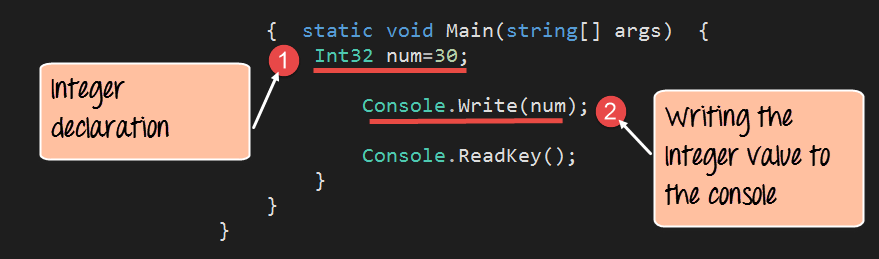```using System;
using System.Collections.Generic;
using System.Linq;
using System.Text;
namespace DemoApplication
{
class Program
{
static void Main(string[] args)
{
Int32 num=30;
Console.Write(num);

}
}
}```

#### Code Explanation:-

1. The Int32 data type is specified to declare an Integer variable called num. The variable is then assigned a value of 30.
2. Finally the console.write function is used to display the number to the console.

If the above code is entered properly and the program is executed successfully, following output will be displayed.

#### Output:From the output, you can clearly see that the Integer variable called num was displayed in the console

### 2) Double

A double data type is used to work with decimals. In this case, the numbers are whole numbers like 10.11, 20.22 or 30.33. In C#, the datatype is denoted by the keyword “Double“. Below is an example of this datatype.

In our example, we will define a double variable called num. We will then assign a Double value to the variable and then display it accordingly.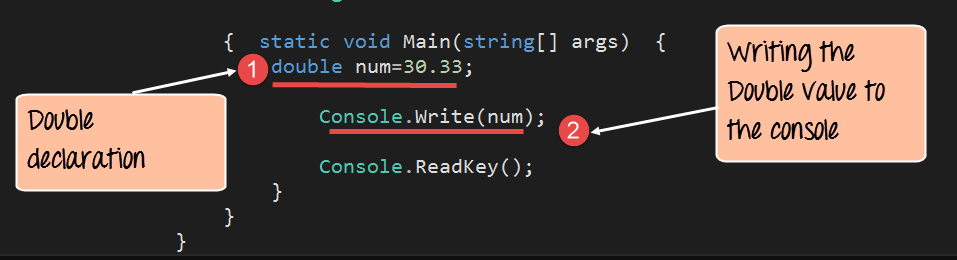```using System;
using System.Collections.Generic;
using System.Linq;
using System.Text;
namespace DemoApplication
{
class Program
{
static void Main(string[] args)
{
double num=30.33;
Console.Write(num);

}
}
}```

#### Code Explanation:-

1. The double data type is specified to declare a double type variable called num. The variable is then assigned a value of 30.33.
2. Finally the console.write function is used to display the number to the console.

If the above code is entered properly and the program is executed successfully, following output will be displayed.

#### Output: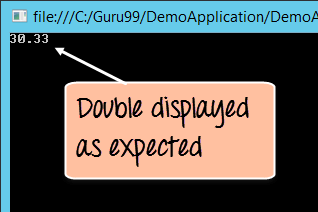From the output, you can clearly see that the double variable called num was displayed in the console

### 3) Boolean

A boolean data type is used to work with Boolean values of true and false. In C#, the datatype is denoted by the Boolean keyword. Below is an example of this datatype can be used.

In our example, we will define a Boolean variable called ‘status.’ We will then assign a boolean value to the variable and then display it accordingly.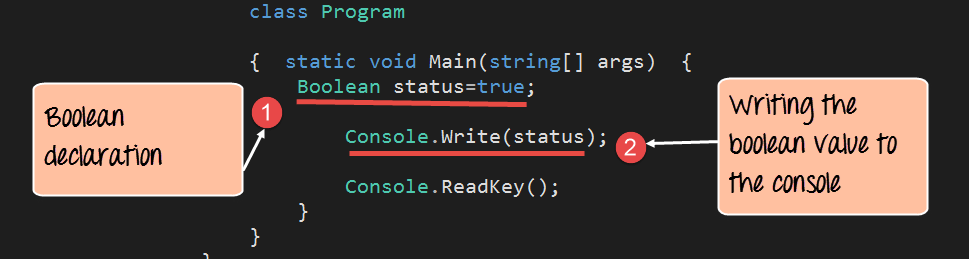```using System;
using System.Collections.Generic;
using System.Linq;
using System.Text;
namespace DemoApplication
{
class Program
{
static void Main(string[] args)
{
Boolean status=true;
Console.Write(status);

}
}
}```

#### Code Explanation:-

1. The boolean data type is specified to declare a Boolean variable called ‘status.’ The variable is then assigned a value of true/false.
2. Finally the console.write function is used to display the Boolean value to the console.

If the above code is entered properly and the program is executed successfully, the output will be displayed.

#### Output:From the output, you can clearly see that the Boolean variable which equals true was displayed in the console

### 4) String

A String data type is used to work with String values. In C#, the datatype is denoted by the keyword ‘String’. Below is an example of this datatype.

In our example, we will define a String variable called ‘message.’ We will then assign a String value to the variable and then display it accordingly.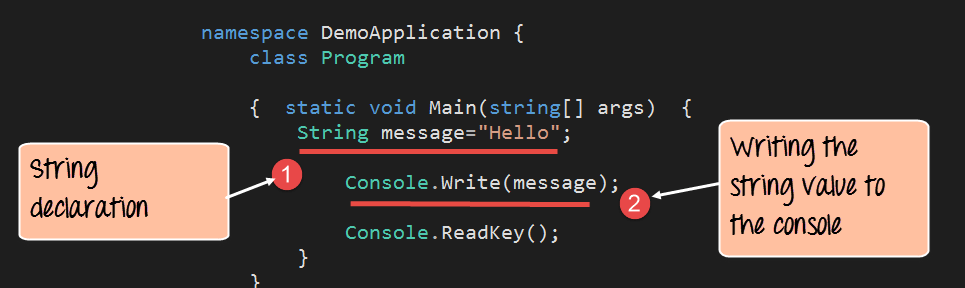```using System;
using System.Collections.Generic;
using System.Linq;
using System.Text;
namespace DemoApplication
{
class program
{
static void Main(string[] args)
{
String message="Hello";
Console.Write(message);

}
}
}```

#### Code Explanation:-

1. The String data type is specified to declare a string variable called message. The variable is then assigned a value of “Hello”.
2. Finally, the console.write function is used to display the string value to the console.

If the above code is entered properly and the program is executed successfully, the output will be displayed.

#### Output: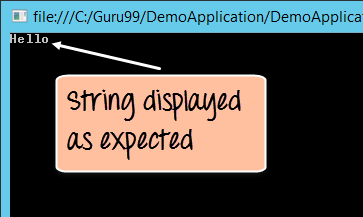From the output, you can clearly see that the String variable called message was displayed in the console